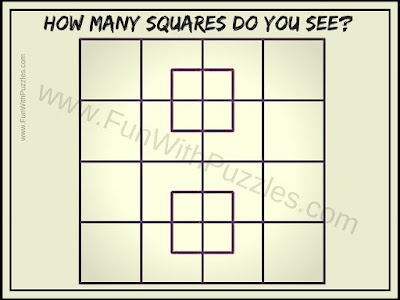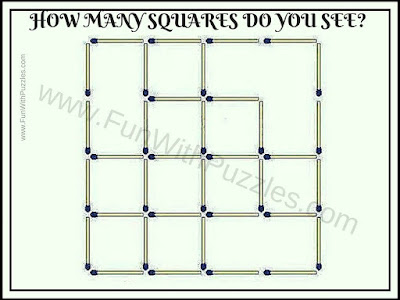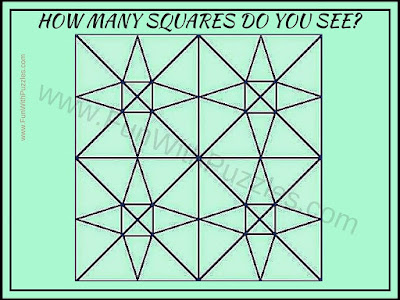Counting the Shapes are very interesting puzzle types. In these types of Picture Puzzles, one has to count the number of a particular shape. Here are 5 such as counting the shapes picture puzzles in which your challenge is to count the number of squares in each of the puzzle pictures. So Can you count correctly the number of squares in each puzzle in your first attempt?1. How many Square can you count?2.  Count the Number of Squares in this picture3. How many squares do you see?4. How Many Squares can you count?5. How Many Squares can you count in this picture?